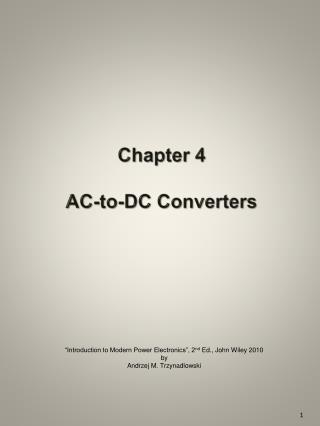DownloadDownload PresentationChapter 4 AC-to-DC Converters

# Chapter 4 AC-to-DC Converters

Download Presentation## Chapter 4 AC-to-DC Converters

- - - - - - - - - - - - - - - - - - - - - - - - - - - E N D - - - - - - - - - - - - - - - - - - - - - - - - - - -
##### Presentation Transcript

1. Chapter 4AC-to-DC Converters “Introduction to Modern Power Electronics”, 2nd Ed., John Wiley 2010 by Andrzej M. Trzynadlowski

2. Three-pulse diode rectifier Fig. 4.1 Chapter 4

3. Example current path and voltage distribution in a three-pulse diode rectifier Fig. 4.2 Chapter 4

4. Waveforms of output voltage and current in a three-pulse diode rectifier (R load) Fig. 4.3 Chapter 4

5. Waveform of input current in a three-pulse diode rectifier (R load) Fig. 4.4 Chapter 4

6. Six-pulse diode rectifier Fig. 4.5 Chapter 4

7. Example current path and voltage distribution in a six-pulse diode rectifier Fig. 4.6 Chapter 4

8. Phasor diagram of voltages in a three-phase ac line, and the conduction area Fig. 4.7 Chapter 4

9. Waveforms of output voltage and current in a six-pulse diode rectifier in the continuous conduction mode (RLE load) Fig. 4.8 Chapter 4

10. Twelve-pulse diode rectifier Fig. 4.9 Chapter 4

11. Areas of conduction modes of a six-pulse diode rectifier Fig. 4.10 Chapter 4

12. Waveforms of output voltage and current in a six-pulse diode rectifier in the discontinuous conduction mode (RLE load) Fig. 4.11 Chapter 4

13. Waveform of input current in a six-pulse diode rectifier (assuming an ideal dc output current) Fig. 4.12 Chapter 4

14. Spectrum of input current in a six-pulse diode rectifier Fig. 4.13 Chapter 4

15. Input filter (harmonic trap) for a three-phase rectifier Fig. 4.14 Chapter 4

16. Phase-controlled (SCR) six-pulse rectifier Fig. 4.15 Chapter 4

17. Firing pulses in a phase-controlled six-pulse rectifier Fig. 4.16 Chapter 4

18. Waveforms of output voltage and current in a phase-controlled six-pulse rectifier in the continuous conduction mode (, RLE load) Fig. 4.17 Chapter 4

19. Control characteristic of a phase-controlled six-pulse rectifier in the continuous conduction mode Fig. 4.18 Chapter 4

20. Rectifier in the inverter mode Fig. 4.19 Chapter 4

21. Waveforms of output voltage and current in a phase-controlled six-pulse rectifier in the continuous conduction mode Fig. 4.20 Chapter 4

22. Area of feasible firing angles Fig. 4.21 Chapter 4

23. Conduction mode areas of a phase-controlled six-pulse rectifier Fig. 4.22 Chapter 4

24. Waveforms of output voltage and current in a phase-controlled six-pulse rectifier in the discontinuous conduction mode: (a) rectifier operation(b) inverter operation Fig. 4.23 Chapter 4

25. Waveform of input current in a phase-controlled six-pulse rectifier (ideal dc output current assumed) Fig. 4.24 Chapter 4

26. Equivalent circuit of a phase-controlled six-pulse rectifier supplied from a practical dc voltage source Fig. 4.25 Chapter 4

27. Waveforms of voltage and current in a phase-controlled six-pulse rectifier during commutation Fig. 4.26 Chapter 4

28. Waveforms of output voltage and current in a phase-controlled six-pulse rectifier supplied from a source with inductance: (a) rectifier mode, (b) inverter mode Fig. 4.27 Chapter 4

29. Notched waveform of input voltage in a phase-controlled six-pulse rectifier supplied from a source with inductance Fig. 4.28 Chapter 4

30. Plane of operation, operating area, and operating quadrants of a rectifier Fig. 4.29 Chapter 4

31. Controlled rectifier with a cross-switch Fig. 4.30 Chapter 4

32. Antiparallel connection of two controlled rectifiers Fig. 4.31 Chapter 4

33. Six-pulse circulating current-free dual converter Fig. 4.32 Chapter 4

34. Six-pulse circulating current-conducting dual converter supplied from two separate ac sources Fig. 4.33 Chapter 4

35. Waveforms of output voltages of constituent rectifiers of a circulating current-conducting dual converter (, ) Fig. 4.34 Chapter 4

36. Waveforms of differential output voltage and circulating current in a circulating current-conducting dual converter () Fig. 4.35 Chapter 4

37. Waveforms of differential output voltage and circulating current in a circulating current-conducting dual converter () Fig. 4.36 Chapter 4

38. Six-pulse circulating current-conducting dual converter supplied from a single ac source Fig. 4.37 Chapter 4

39. Single-phase PWM rectifier with an LC input filter Fig. 4.38 Chapter 4

40. Switching pattern of a hypothetical four-state PWM converter Fig. 4.39 Chapter 4

41. Stator of a three-phase electric ac machine Fig. 4.40 Chapter 4

42. Generation of a space vector of the stator MMFs in a three-phase electric ac machine: (a) phasor diagram of stator currents, (b) vectors of MMFs Fig. 4.41 Chapter 4

43. Space vector of stator MMFs and its components Fig. 4.42 Chapter 4

44. Synthesis of a rotating space vector from stationary vectors and Fig. 4.43 Chapter 4

45. Voltage space vector in the stationary and rotating reference frames Fig. 4.44 Chapter 4

46. Current-type PWM rectifier Fig. 4.45 Chapter 4

47. Reference current vector in the vector space of input currents of a current-type PWM rectifier Fig. 4.46 Chapter 4

48. Example waveforms of switching variables in one switching cycle of a current-type PWM rectifier Fig. 4.47 Chapter 4

49. Control scheme of a current-type PWM rectifier Fig. 4.48 Chapter 4

50. Waveforms of output voltage and current in a current-type PWM rectifier: (a) m = 0.75, (b) m = 0.35 (fsw/fo = 24, RLE load) Fig. 4.49 Chapter 4